Continuous Wavelet Transform and Scale-Based Analysis

Definition of the Continuous Wavelet Transform

Like the Fourier transform, the continuous wavelet transform (CWT) uses inner products to measure the similarity between a signal and an analyzing function. In the Fourier transform, the analyzing functions are complex exponentials, ${e}^{j\omega t}$. The resulting transform is a function of a single variable, ω. In the short-time Fourier transform, the analyzing functions are windowed complex exponentials, $w\left(t\right){e}^{j\omega t}$, and the result is a function of two variables. The STFT coefficients, $F\left(\omega ,\tau \right),$ represent the match between the signal and a sinusoid with angular frequency ω in an interval of a specified length centered at τ.

In the CWT, the analyzing function is a wavelet, ψ. The CWT compares the signal to shifted and compressed or stretched versions of a wavelet. Stretching or compressing a function is collectively referred to as dilation or scaling and corresponds to the physical notion of scale. By comparing the signal to the wavelet at various scales and positions, you obtain a function of two variables. The 2-D representation of a 1-D signal is redundant. If the wavelet is complex-valued, the CWT is a complex-valued function of scale and position. If the signal is real-valued, the CWT is a real-valued function of scale and position. For a scale parameter, a>0, and position, b, the CWT is:

$C\left(a,b;f\left(t\right),\psi \left(t\right)\right)={\int }_{-\infty }^{\infty }f\left(t\right)\frac{1}{a}{\psi }^{*}\left(\frac{t-b}{a}\right)dt$

where $*$ denotes the complex conjugate. Not only do the values of scale and position affect the CWT coefficients, but the choice of wavelet also affects the values of the coefficients.

By continuously varying the values of the scale parameter, a, and the position parameter, b, you obtain the cwt coefficients C(a,b). Note that for convenience, the dependence of the CWT coefficients on the function and analyzing wavelet has been suppressed.

Multiplying each coefficient by the appropriately scaled and shifted wavelet yields the constituent wavelets of the original signal.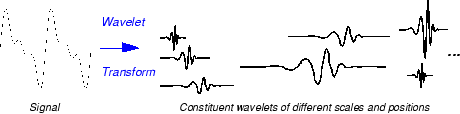There are many different admissible wavelets that can be used in the CWT. While it may seem confusing that there are so many choices for the analyzing wavelet, it is actually a strength of wavelet analysis. Depending on what signal features you are trying to detect, you are free to select a wavelet that facilitates your detection of that feature. For example, if you are trying to detect abrupt discontinuities in your signal, you may choose one wavelet. On the other hand, if you are interested in finding oscillations with smooth onsets and offsets, you are free to choose a wavelet that more closely matches that behavior.

Scale

Like the concept of frequency, scale is another useful property of signals and images. For example, you can analyze temperature data for changes on different scales. You can look at year-to-year or decade-to-decade changes. Of course, you can examine finer (day-to-day), or coarser scale changes as well. Some processes reveal interesting changes on long time, or spatial scales that are not evident on small time or spatial scales. The opposite situation also happens. Some of our perceptual abilities exhibit scale invariance. You recognize people you know regardless of whether you look at a large portrait, or small photograph.

To go beyond colloquial descriptions such as “stretching” or “shrinking” we introduce the scale factor, often denoted by the letter a. The scale factor is an inherently positive quantity, a>0. For sinusoids, the effect of the scale factor is very easy to see.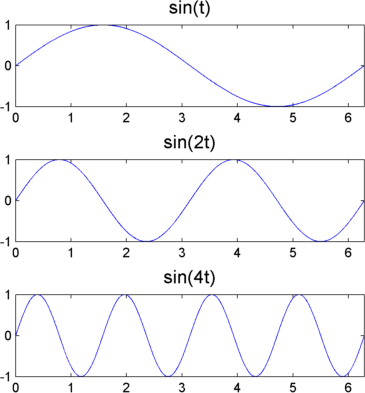In sin(at), the scale is the inverse of the radian frequency, a.

The scale factor works exactly the same with wavelets. The smaller the scale factor, the more “compressed” the wavelet. Conversely, the larger the scale, the more stretched the wavelet. The following figure illustrates this for wavelets at scales 1,2, and 4.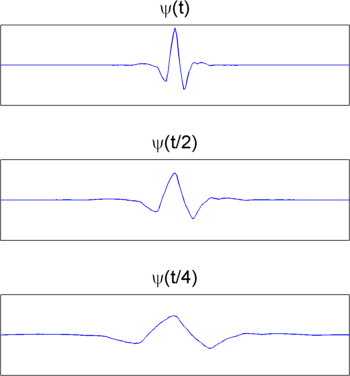This general inverse relationship between scale and frequency holds for signals in general.

Not only is a time-scale representation a different way to view data, but it also is a very natural way to view data derived from a great number of natural phenomena.

Scale and Frequency

There is clearly a relationship between scale and frequency. Recall that longer scales correspond to the most “stretched” wavelets. The more stretched the wavelet, the longer the portion of the signal with which it is being compared, and therefore the coarser the signal features measured by the wavelet coefficients.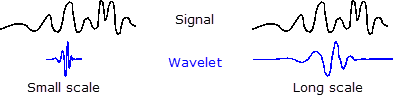To summarize, the general correspondence between scale and frequency is:

• Small scale a ⇒ Compressed wavelet ⇒ Rapidly changing details ⇒ High frequency ω.

• Long scale a ⇒ Stretched wavelet ⇒ Slowly changing, coarse features ⇒ Low frequency ω.

While there is a general relationship between scale and frequency, no precise relationship exists. Users familiar with Fourier analysis often want to define a mapping between a wavelet at a given scale with a specified sampling period to a frequency in hertz. You can only do this in a general sense. Therefore, it is better to talk about the pseudo-frequency corresponding to a scale. The Wavelet Toolbox™ software provides two functions centfrq and scal2frq, which enable you to find these approximate scale-frequency relationships for specified wavelets and scales.

The basic approach identifies the peak power in the Fourier transform of the wavelet as its center frequency and divides that value by the product of the scale and the sampling interval. See scal2frq for details. The following example shows the match between the estimated center frequency of the db8 wavelet and a sinusoid of the same frequency.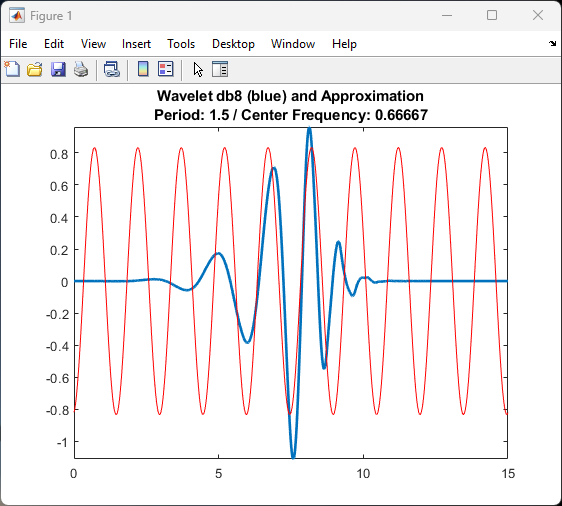The relationship between scale and frequency in the CWT is also explored in Continuous Wavelet Transform as a Bandpass Filter.

Shifting

Shifting a wavelet simply means delaying (or advancing) its onset. Mathematically, delaying a function f(t) by k is represented by f(tk):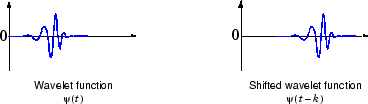CWT as a Windowed Transform

In Short-Time Fourier Transform, the STFT is described as a windowing of the signal to create a local frequency analysis. A shortcoming of the STFT approach is that the window size is constant. There is a trade off in the choice of window size. A longer time window improves frequency resolution while resulting in poorer time resolution because the Fourier transform loses all time resolution over the duration of the window. Conversely, a shorter time window improves time localization while resulting in poorer frequency resolution.

Wavelet analysis represents the next logical step: a windowing technique with variable-sized regions. Wavelet analysis allows the use of long time intervals where you want more precise low-frequency information, and shorter regions where you want high-frequency information.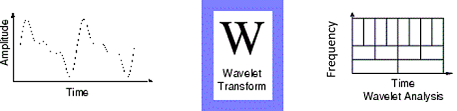The following figure contrasts the different ways the STFT and wavelet analysis decompose the time-frequency plane.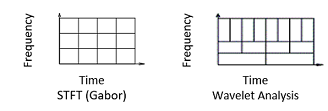Wavelet Toolbox Documentation평가판 신청